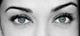# Math Is Fun Forum

Discussion about math, puzzles, games and fun.   Useful symbols: ÷ × ½ √ ∞ ≠ ≤ ≥ ≈ ⇒ ± ∈ Δ θ ∴ ∑ ∫  π  -¹ ² ³ °

You are not logged in.

## #1 2006-12-14 06:09:02

unique
MemberRegistered: 2006-10-04
Posts: 419

### equation of line

Find the equation of the line with a slope of -3/4 and a y-intercept of -3

i did:

slope intercept equation of the line whose slope is -3/4 and whose y intercept is -3 is
y = -3/4x +(-3)

Desi
Raat Key Rani !

Offline

## #2 2006-12-14 07:20:14

luca-deltodesco
MemberRegistered: 2006-05-05
Posts: 1,470

### Re: equation of line

that is correct, although you would probably write it as

y = -3/4x - 3 or y + 3/4x + 3 = 0, or 4y + 3x + 12 = 0

The Beginning Of All Things To End.
The End Of All Things To Come.

Offline